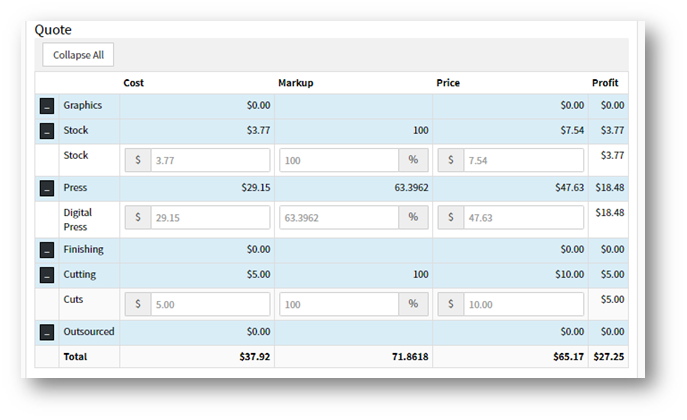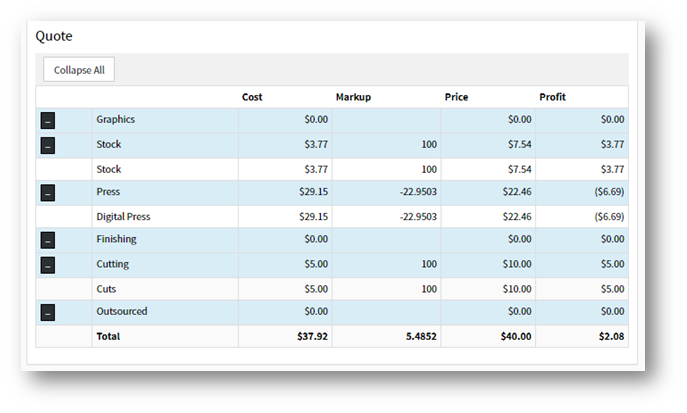On the Quote table of an item, a negative value may appear under Press in the pricing breakdown. A negative value will appear as (\$___) in the profit column for Press.This negative value normally stems from Template Option pricing being utilized where the calculated price is lower than the Calculate By Press price. When this happens, the difference gets applied to the press.

Example 1:

Calculate by Template Option price is lower than Calculate by Press price.

 Calculate by Press Calculate by TemplateExample 2:

Calculate by Press vs. Calculate by Template Option with a Department Item.

The Template Option price is set at \$60. Due to the department item being included in the job calculation price, the pricing needed to be adjusted by \$17.46. That value was added into the press price.

 Calculate by Press Calculate by Template with Department Item included in Job Calculation Price Calculate by Template without Department Item included in Job Calculation Price